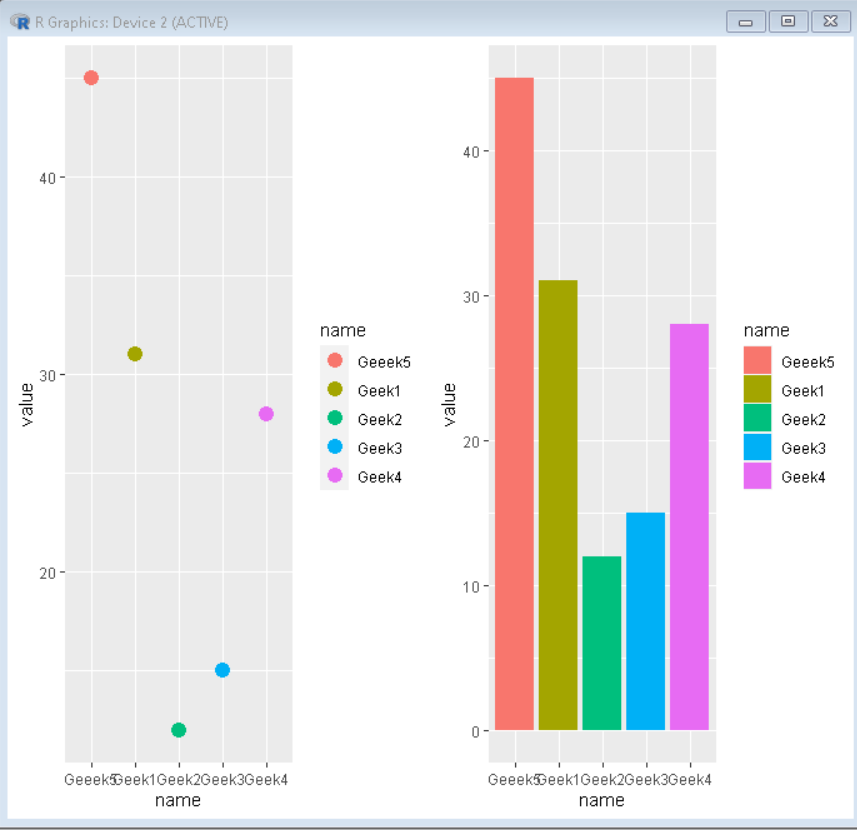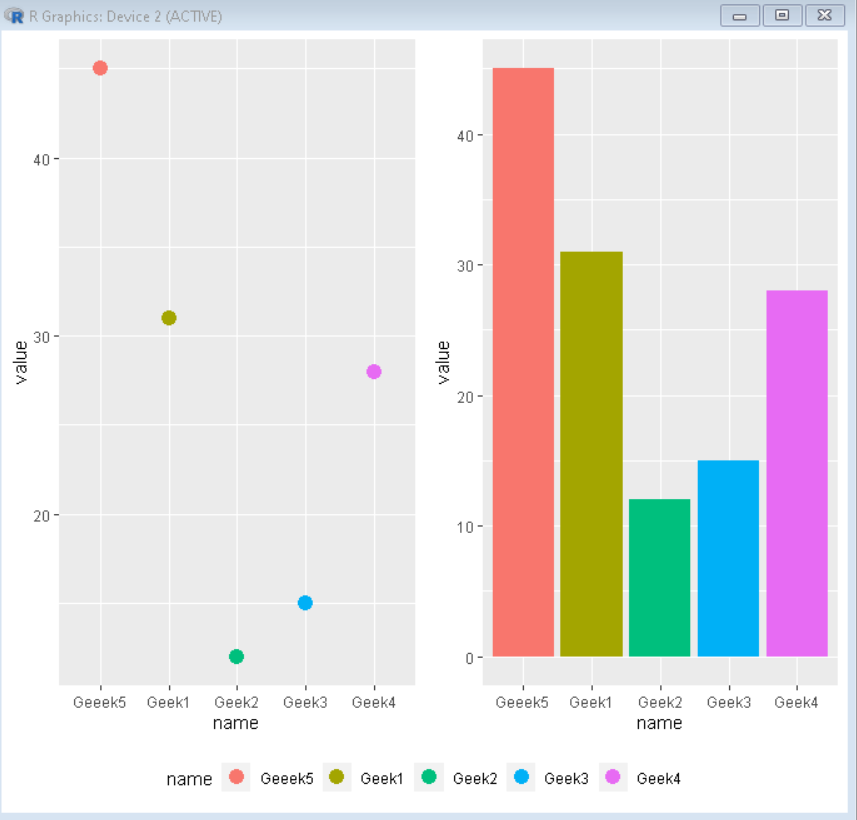# Add Common Legend to Combined ggplot2 Plots in R

In this article, we will discuss how to create a combined plot with a shared legend in R Language using the ggplot2 package.

To join multiple ggplot2 plots, we use the grid.arrange() function of the gridExtra package in the R Language. The grid.arrange() function converts the frame into a grid of the desired number of rows and columns. Then we put different plots in different parts of the grid to create the desired look for multiple plots all in the same frame.

Syntax:

grid.arrange( plot1, plot2,……, ncol/nrow)

where,

1. ncol: Determines the number of columns in the grid.
2. nrow: Determines the number of rows in the grid.

Example:

Here, is a combined plot made using the grid.arrange() function of the ggplot2 package.

## R

 `# Load ggplot2 and gridExtra ` `library``(``"ggplot2"``)  ` `library``(``"gridExtra"``) ` ` `  `# Create sample data ` `set.seed``(5642)                              ` `sample_data <- ``data.frame``( ` `  ``name = ``c``(``"Geek1"``,``"Geek2"``,``"Geek3"``, ` `           ``"Geek4"``,``"Geeek5"``) , ` `  ``value=``c``(31,12,15,28,45)) ` ` `  ` `  `# Create both plot and store in variable ` `plot1<-``ggplot``(sample_data, ` `              ``aes``(x = name, y = value, col=name)) + ` `              ``geom_point``(size=4) ` `plot2<-``ggplot``(sample_data, ` `              ``aes``(x = name, y = value, fill=name)) + ` `              ``geom_col``() ` ` `  `# combine both plots using grid.arrange() ` `grid.arrange``(plot1, plot2, ncol = 2)`

Output:## Combined plot with shared legend

To create a combined plot with shared legend we follow the following steps:

Step 1: Create a combined plot using the grid.arrange() function without any legend.

## R

 `# To remove legend ` `plot+ ``theme``( legend.position = ``"none"` `)  ` ` `  `# arrange side by side in a grid ` `grid.arrange``( plot1 , plot2 , ncol=2)`

Step 2: Create a function that extracts legend out of the ggplot2 plot and returns it as a ggplot element.

## R

 `get_only_legend <- ``function``(plot) { ` `   `  `# get tabular interpretation of plot ` `plot_table <- ``ggplot_gtable``(``ggplot_build``(plot))  ` `   `  `#  Mark only legend in plot ` `legend_plot <- ``which``(``sapply``(plot_table\$grobs, ``function``(x) x\$name) == ``"guide-box"``)  ` `                             `  `# extract legend ` `legend <- plot_table\$grobs[[legend_plot]] ` `                             `  `# return legend ` `return``(legend)  ` `}`

Step3: Combine legend obtained from the above function using the grid.arrange() function.

## R

 `grid.arrange``(combined_plot, legend, nrow = 2, heights = ``c``(10, 1))`

And the resulting plot will be a combined plot with a shared legend.

Example:

Here, is a combined plot made using the grid.arrange() function with shared legend.

## R

 `# Create sample data ` `set.seed``(5642)                              ` `sample_data <- ``data.frame``( ` `  ``name = ``c``(``"Geek1"``,``"Geek2"``,``"Geek3"``, ` `           ``"Geek4"``,``"Geeek5"``) , ` `  ``value=``c``(31,12,15,28,45)) ` ` `  `# Load ggplot2 and gridExtra ` `library``(``"ggplot2"``)  ` `library``(``"gridExtra"``) ` ` `  `# Create both plot and store in variable without legend ` `plot1<-``ggplot``(sample_data, ` `              ``aes``(x = name, y = value, col=name)) + ` `              ``geom_point``(size=4)+ ` `              ``theme``(legend.position = ``"none"``) ` `plot2<-``ggplot``(sample_data, ` `              ``aes``(x = name, y = value, fill=name)) + ` `              ``geom_col``()+ ` `              ``theme``(legend.position = ``"none"``) ` ` `  `# combine both plots using grid.arrange() ` `combined_plot <- ``grid.arrange``(plot1, plot2, ncol = 2) ` ` `  `# plot1 with legend ` `plot1_legend <- ``ggplot``(sample_data, ` `              ``aes``(x = name, y = value, col=name)) + ` `              ``geom_point``(size=4)+ ` `              ``theme``(legend.position = ``"bottom"``) ` ` `  `# function to extract legend from plot ` `get_only_legend <- ``function``(plot) { ` `  ``plot_table <- ``ggplot_gtable``(``ggplot_build``(plot)) ` `  ``legend_plot <- ``which``(``sapply``(plot_table\$grobs, ``function``(x) x\$name) == ``"guide-box"``) ` `  ``legend <- plot_table\$grobs[[legend_plot]] ` `  ``return``(legend) ` `} ` `                           `  `# extract legend from plot1 using above function ` `legend <- ``get_only_legend``(plot1_legend)    ` `    `  `# final combined plot with shared legend ` `grid.arrange``(combined_plot, legend, nrow = 2, heights = ``c``(10, 1))`

Output:Whether you're preparing for your first job interview or aiming to upskill in this ever-evolving tech landscape, GeeksforGeeks Courses are your key to success. We provide top-quality content at affordable prices, all geared towards accelerating your growth in a time-bound manner. Join the millions we've already empowered, and we're here to do the same for you. Don't miss out - check it out now!